## Thursday, September 1, 2011

### Modern Portfolio Theory - A Python Implementation

I was surprised last week to find there was no accessible Python implementation of the calculation of the Efficient Frontier (as defined by Markowitz in his presentation of Modern Portfolio Theory ~1957).* Since the problem seemed simple to solve with the tools at hand, I set out to "right the wrong" and develop an open implementation (available under an open, BSD license via github) that meets my needs.

In the process of building the basic metrics, I was able to experiment with NumPy's datetime support -- as mentioned in my earlier post. The API's are still a bit in flux, but I thought I would write up a full workflow using the tools as they are. I would greatly appreciate feedback/contributions/suggestions.  So let's dive in ...

Modern Portfolio Theory
While there are much better sources for an explanation of MPT, I'll take a stab at a high level introduction to the basic concepts. MPT is based on the idea that a diversified portfolio--a portfolio that holds several assets, or asset classes, that have some inverse correlation--may be constructed which provides less risk and the same return as any of the single assets.  Or similarly, an asset mix may be chosen that has the same risk as any single asset, but a higher return. If one plots the returns of an asset along the value (y) axis versus the risk of an asset along the index (x) axis, it's easy to spot desirable assets based on their location on the chart.  While an efficient market may imply that there is a close correlation between risk and return, some assets may lie closer to the upper left portion of the chart.  These would provide less risk and higher returns than assets falling in the lower right portion of the chart.

Figure: 1 Plot of Risk (Annual Volatility: x axis) vs. Return (Annualized Returns: y axis)

For example, it's easy to see in Figure 1 that BA might be a less preferable asset than COP, since COP has a higher return for less risk (historically, at least).

While there are acknowledged problems with using historical standard deviation as a proxy for risk, we'll continue to implement the standard model for now.  In this standard model for MPT, one may construct a portfolio, by holding assets in optimal quantities--or relative weights--that lies along a line of all optimal portfolios, or along the Efficient Frontier.

Points along the Efficient Frontier may be found by solving an optimization problem which finds, for example, the best weighting of assets to maximize return for a given risk tolerance. This is the approach I take. The mpt.py module closely follows the gradient method described by William Sharpe to accomplish this.

The Software Model
While I've tried to keep things fairly functionally oriented, I have created two objects that accomplish the tasks of managing the data structures and providing methods. The first object is defined by the Stock class. It has methods to load data and to store metrics about an asset.  The metrics are populated when the class calls metrics functions from the metrics module on its data.

Before I use my Stock object, I'll start from scratch by pre-populating a sqlite database file with some useful price data. You only have to do this once, or as many times as you want to keep your data fresh:

Notice that I've loaded symbols from a csv file (available in the github repo) that has a short sampling of assets from the S&P 500. (There is also a complete list of S&P symbols in the repo). The populate_db method iterates over these symbols and pulls the price data from Yahoo Finance. Not all the assets loaded from Yahoo (I may have some symbol issues), but I got what I could. An additional asset's price data is also pulled down to be used as a benchmark. "^GSPC" is the symbol for the S&P 500 index itself. Note that passing a list or a filename as the symbols argument both work.

Now, on to an example session using a Stock object:

This is pretty straightforward. A Stock object and its metrics are generated, as expected. Things begin to get interesting when the other object in the MPT workflow is introduced: the Portfolio object. As one might guess, it contains a collection of Stock objects. Each Stock object has had its ratearray appropriately truncated and imputed in order for every asset's data length to match.

Here's an example of its usage:

Notice that the recommended allocation for a risk tolerance of 2.0 has AAPL weighted to 100% of the portfolio. This indicates that AAPL is actually on the Efficient Frontier for the given asset options--i.e. there is no combination of assets that gives the same return for less risk. When the risk tolerance is reduced to 0.5, the allocations include KO as well -- reducing the risk and return, and revealing another point on the Frontier. So, let's take a look at how the calculations were implemented in Python.

At a high level, the approach for the optimization is to begin with equal weights of all assets. The marginal utility of all the assets is then calculated and used to determine an optimal two-asset swap. The process is repeated until two-asset swaps result in minimal (below a threshold) improvement. This is done within the confines of the upper and lower bounds specifically imposed on our weightings.

Marginal Utility
The marginal utility calculation is given by: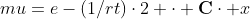Where: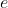: Vector of expected return of assets. Uses annualized_adjusted_return for each asset.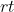: Scalar value of risk tolerance in daily volatility.: Covariance matrix of assets in portfolio.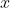: Current portfolio weighting.

For example, the marginal utility in the portfolio we created earlier can be gotten by:

This is interpreted as: the utility for AAPL increases at a rate of 33.19% for every unit change in the amount invested in AAPL.

Two Asset Swap
Taking the min and max of the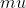matrix elements reveals which two assets to swap, and in which direction. What remains is to calculate the optimal amount to swap. This is given by: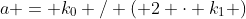Where: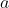: The optimal amount to swap.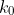: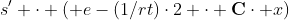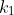: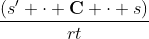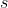: A vector representing the stocks to be swapped. For our example, this looks like: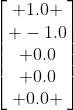I'll leave the derivation to William Sharpe. The implementation, given SciPy's and NumPy's tool set and expressiveness, was quite simple. Though the bounds checking is a bit of sausage making, the actual methods on the portfolio almost write themselves.

All this is nice algorithmically, but sometimes it helps to have some graphical interaction, and a visualization of what's happening. This is where the Enthought Tool Suite (ETS) came in handy.

A GUI App
Up to this point the only dependencies for the MPT workflow are SQLite, NumPy, and SciPy. To get a visualization, we use a separate module (chaco_mpt_display.py) to achieve coolness. We do, however, add the full stack of Enthought tools as a dependency for this module. The good news is that all dependencies can be easily gotten with the Enthought Python Distribution. Below is a quick video of this afternoon's version of the GUI--it's changing fairly rapidly as I pile in new features.

Any feedback or corrections are welcome -- feel free to get the code from github. Pull requests are welcome!

* I recently found a nascent implementation that is fairly tied to a visualization -- I like my approach much better.

1.Nice! What element of Chaco does allow you to drag'n'drop the labels of the data points? Is that part of chaco_mpt_display?

2.Chaco's DataLabel does this out of the box for the (x, y) values. I did have to subclass this to get support for arbitrary text in the labels. (see data_point_label.py in the github repo).

3.The CVXOPT documentation has a portfolio optimization example:

http://abel.ee.ucla.edu/cvxopt/examples/book/portfolio.html

It's also fairly easy to do Sharpe ratio optimization with CVXOPT's quadratic programming function.

4.You may be interested in a book called "Stochastic Portfolio Theory" by Dr. Fernholz.

5.I believe you are daily-ifying your volatility incorrectly. You start with rt = 0.5, and say that represents a 50% annual volatility. That's fine. But then you calculate the daily volatility as drt = rt / 252.0. Since volatility scales with the square root of time, it should be drt = rt / math.sqrt(252.0). If you wanted rt and drt to be variance (i.e. the square of volatility), then you could just divide by 252, but that isn't what you say and isn't likely what you meant. Hope this helps.

6.@JB Thanks for pointing this out...I'll take a look and follow up later.

7.Amazing Post! Amazing Bloq ! Friends and me are currently very interested in developing something close to this CAPM Project, Travis. Have you already implemented Mikes suggestion of a optimazation or a more recent version? Best wishes from Germany.